Main MRPT website > C++ reference for MRPT 1.5.7
2D/3D point and pose PDFs

## Detailed Description

Collaboration diagram for 2D/3D point and pose PDFs: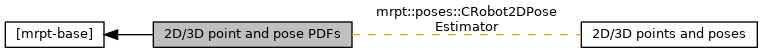## Classes

class  mrpt::poses::CPoint2DPDF
Declares a class that represents a Probability Distribution function (PDF) of a 2D point (x,y). More...

class  mrpt::poses::CPoint2DPDFGaussian
A gaussian distribution for 2D points. More...

class  mrpt::poses::CPointPDF
Declares a class that represents a Probability Distribution function (PDF) of a 3D point (x,y,z). More...

class  mrpt::poses::CPointPDFGaussian
A gaussian distribution for 3D points. More...

class  mrpt::poses::TSimple3DPoint
Data within each particle. More...

class  mrpt::poses::CPointPDFParticles
A probability distribution of a 2D/3D point, represented as a set of random samples (particles). More...

class  mrpt::poses::CPointPDFSOG
Declares a class that represents a Probability Density function (PDF) of a 3D point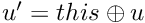. More...

class  mrpt::poses::CPose2DGridTemplate< T >
This is a template class for storing a 3D (2D+heading) grid containing any kind of data. More...

class  mrpt::poses::CPose3DPDF
Declares a class that represents a Probability Density Function (PDF) of a 3D pose (6D actually). More...

class  mrpt::poses::CPose3DPDFGaussian
Declares a class that represents a Probability Density function (PDF) of a 3D pose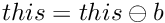. More...

class  mrpt::poses::CPose3DPDFGaussianInf
Declares a class that represents a Probability Density function (PDF) of a 3D poseas a Gaussian described by its mean and its inverse covariance matrix. More...

class  mrpt::poses::CPose3DPDFParticles
Declares a class that represents a Probability Density function (PDF) of a 3D pose. More...

class  mrpt::poses::CPose3DPDFSOG
Declares a class that represents a Probability Density function (PDF) of a 3D(6D) pose. More...

class  mrpt::poses::CPose3DQuatPDF
Declares a class that represents a Probability Density Function (PDF) of a 3D pose (6D actually), by means of a 7-vector with a translation [x y z] and a quaternion [qr qx qy qz]. More...

class  mrpt::poses::CPose3DQuatPDFGaussian
Declares a class that represents a Probability Density function (PDF) of a 3D pose using a quaternion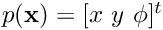. More...

class  mrpt::poses::CPose3DQuatPDFGaussianInf
Declares a class that represents a Probability Density function (PDF) of a 3D pose using a quaternion. More...

class  mrpt::poses::CPosePDF
Declares a class that represents a probability density function (pdf) of a 2D pose (x,y,phi). More...

class  mrpt::poses::CPosePDFGaussian
Declares a class that represents a Probability Density function (PDF) of a 2D pose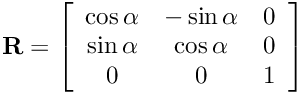. More...

class  mrpt::poses::CPosePDFGaussianInf
A Probability Density function (PDF) of a 2D poseas a Gaussian with a mean and the inverse of the covariance. More...

class  mrpt::poses::CPosePDFGrid
Declares a class that represents a Probability Distribution function (PDF) of a 2D pose (x,y,phi). More...

class  mrpt::poses::CPosePDFParticles
Declares a class that represents a Probability Density Function (PDF) over a 2D pose (x,y,phi), using a set of weighted samples. More...

class  mrpt::poses::CPosePDFSOG
Declares a class that represents a Probability Density function (PDF) of a 2D pose. More...

class  mrpt::poses::CPoseRandomSampler
An efficient generator of random samples drawn from a given 2D (CPosePDF) or 3D (CPose3DPDF) pose probability density function (pdf). More...

class  mrpt::poses::CRobot2DPoseEstimator
A simple filter to estimate and extrapolate the robot 2D (x,y,phi) pose from asynchronous odometry and localization/SLAM data. More...

## Namespaces

mrpt::poses
Classes for 2D/3D geometry representation, both of single values and probability density distributions (PDFs) in many forms.

 Page generated by Doxygen 1.8.14 for MRPT 1.5.7 Git: 8277875f6 Mon Jun 11 02:47:32 2018 +0200 at lun oct 28 01:50:49 CET 2019